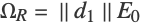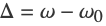# The AC Stark Effect

A formula for the energy shifts due to an optical field is obtained for a two-level atomic system.

We define an atomic system consisting of two states (a ground state labeled 1 and upper state labeled 2). This is a "toy" system that neglects angular momentum (J and M are not defined). We apply a light field detuned from resonance by a frequency Δ.

 Define the atomic system.
 Define the optical field with frequency ω. This is an x polarized field by default, but for a toy system the polarization does not matter.
 The Hamiltonian for the system subject to the optical field. For simplicity of notation, we define the "reduced" Rabi frequency.
 Assuming that, we can apply the rotating-wave approximation to the Hamiltonian. We define the detuning.

The energy eigenvalues are found by diagonalizing the Hamiltonian.

 The energy eigenvalues in the presence of the optical field.
 Plot the eigenvalues as a function of Rabi frequency.

To find the Stark shifts, we subtract the eigenenergies for zero applied field. This gives one shift that is always positive, and one that is negative. (This is because the eigenvalue ordering changes as Δ goes through zero.) To obtain the desired physical behavior, in which the energy shifts flip sign as Δ goes through zero, we multiply by Δ/(equivalent to Sign[Δ]).

 Calculate the energy shifts.
 A plot of the energy shift for the excited state as a function of detuning.
 To lowest order, the shifts are quadratic.

Note that these solutions lose validity as. This is because we have neglected the natural line width of the upper state. When the detuning is on the order of or smaller than the natural line width, the formula must be modified. To analyze the general case, we can model the effect of the upper-state line width Γ by making the upper-state energy complex.

 Add an imaginary part to the upper-state energy.
 Find the eigenvalues of the modified Hamiltonian.

The energies are the real parts of the eigenvalues of the modified Hamiltonian. In order to find compact expressions for the real parts, it helps to do a little manipulation by hand.

 Add Δ/2 to each eigenvalue and take the real part.
 The energies are now equal in magnitude and opposite in sign. Square each and simplify.
 Reverse the procedure by taking the square root, restoring the opposite signs, and subtracting Δ/2.
 Again, we calculate the energy shifts by subtracting the zero-field energies and multiplying by Sign[Δ].
 The shift now turns around and goes through zero as the field goes on resonance.
 A plot of the upper-state shift as a function of Rabi frequency and detuning.

To lowest order in the Rabi frequency the shifts are given by dispersive Lorentzian functions.